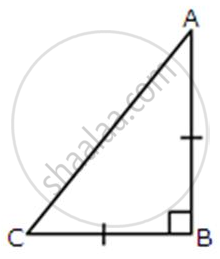# ABC is an isosceles right-angled triangle. Assuming of AB = BC = x, find the value of each of the following trigonometric ratio: cos 45° - Mathematics

Sum

ABC is an isosceles right-angled triangle. Assuming of AB = BC = x, find the value of each of the following trigonometric ratio: cos 45°#### Solution

Given that AB = BC = x

∴ AC = sqrt(AB^2+BC^2) = sqrt(x^2 + x^2) = xsqrt2

cos 45° ="BC"/"AC" = x/(xsqrt2) = 1/sqrt2

Concept: Trigonometric Ratios of Some Special Angles
Is there an error in this question or solution?

#### APPEARS IN

Selina Concise Mathematics Class 9 ICSE
Chapter 23 Trigonometrical Ratios of Standard Angles [Including Evaluation of an Expression Involving Trigonometric Ratios]
Exercise 23 (A) | Q 5.2 | Page 291